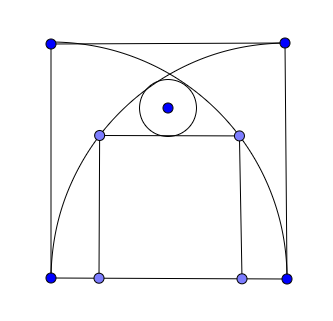# Yeah! I reached 200,000 points

Geometry Level 5As shown in the figure above, two quarter circles are inscribed in a square of side length 5. A smaller square is inscribed in between the two quarter circles and a circle of radius $r$ is inscribed in between the smaller square and the two quarter circles. What is $r$?

×